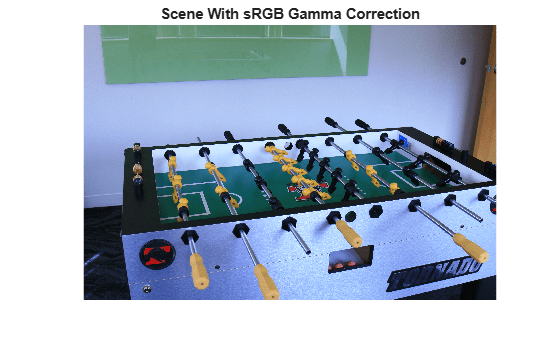rgb2lin

Linearize gamma-corrected RGB values

Description

B = rgb2lin(A) undoes the gamma correction of the sRGB values in image A so that B contains linear RGB values.

example

B = rgb2lin(A,Name,Value) undoes gamma correction using name-value pairs to control additional options.

Examples

collapse all

Open an image. The JPEG file format saves images in the gamma-corrected sRGB color space.

Display the image.

imshow(A)
title('Scene With sRGB Gamma Correction')Undo the gamma correction and linearize the image by using the rgb2lin function. Optionally, specify the data type of the linearized values.

B = rgb2lin(A,'OutputType','double');

Display the linearized image. Shadows in the linearized image are darker than in the original image, as expected.

imshow(B)
title('Scene Without sRGB Gamma Correction')Input Arguments

collapse all

Gamma-corrected RGB color values, specified as a numeric array in one of the following formats.

• c-by-3 colormap. Each row specifies one RGB color value.

• m-by-n-by-3 image

• m-by-n-by-3-by-p stack of images

Data Types: single | double | uint8 | uint16

Name-Value Arguments

Specify optional comma-separated pairs of Name,Value arguments. Name is the argument name and Value is the corresponding value. Name must appear inside quotes. You can specify several name and value pair arguments in any order as Name1,Value1,...,NameN,ValueN.

Example: B = lin2rgb(I,'ColorSpace','adobe-rgb-1998') linearizes the gamma-corrected image, I, according to the Adobe RGB (1998) standard.

Color space of the input image, specified as the comma-separated pair consisting of 'ColorSpace' and 'srgb' or 'adobe-rgb-1998'.

Data Types: char | string

Data type of the output RGB values, specified as the comma-separated pair consisting of 'OutputType' and 'double', 'single', 'uint8', or 'uint16'. By default, the output data type is the same as the data type of A.

Data Types: char | string

Output Arguments

collapse all

Linearized RGB color values, returned as a numeric array of the same size as the input A.

Algorithms

collapse all

Linearization Using the sRGB Standard

sRGB tristimulus values are linearized using the following parametric curve:

f(u) = -f(-u),                u < 0

f(u) = cu,                0 ≤ u < d

f(u) = (au + b)ɣ,      ud,

where u represents a color value with these parameters:

a = 1/1.055

b = 0.055/1.055

c = 1/12.92

d = 0.04045

ɣ = 2.4

Linearization Using the Adobe RGB (1998) Standard

Adobe RGB (1998) tristimulus values are linearized using a simple power function:

v = uɣ,

with

ɣ = 2.19921875

 Ebner, Marc. "Gamma Correction." Color Constancy. Chichester, West Sussex: John Wiley & Sons, 2007.

 Adobe Systems Incorporated. "Inverting the color component transfer function." Adobe RGB (1998) Color Image Encoding. Section 4.3.5.2, May 2005, p.12.